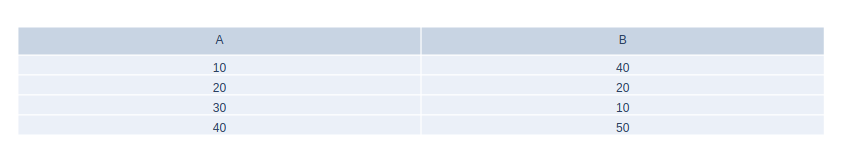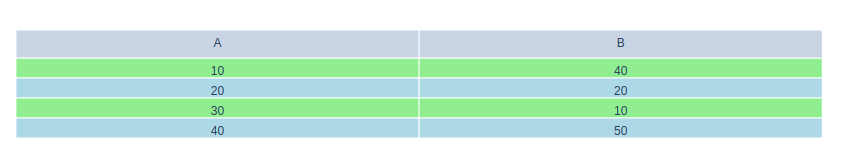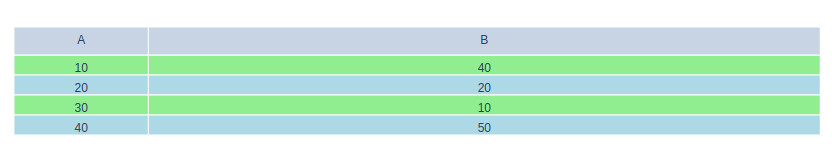Open In App

# How to create Tables using Plotly in Python?

Plotly is a Python library that is used to design graphs, especially interactive graphs. It can plot various graphs and charts like histogram, barplot, boxplot, spreadplot, and many more. It is mainly used in data analysis as well as financial analysis. plotly is an interactive visualization library.

## Tables in Plotly

A table helps in organizing the data into columns and rows. The use of tables is prevalent throughout all communication, research, and data analysis.  They privilege rapid entrance and proportionately elementary comparison of information. It can be created using the Table() method of graph_objects class.

Syntax: plotly.graph_objects.Table(arg=None, cells=None, columnorder=None, columnwidth=None, header=None, **kwargs)

Parameters:

arg:  dict of properties compatible with this constructor or an instance of plotly.graph_objects.Table

cells: plotly.graph_objects.table.Cells instance or dict with compatible properties

columnorder: Specifies the rendered order of the data columns; for example, a value 2 at position 0 means that column index 0 in the data will be rendered as the third column, as columns have an index base of zero.

columnwidth: The width of columns expressed as a ratio. Columns fill the available width in proportion of their specified column widths.

Example:

## Python3

 `import` `plotly.graph_objects as go`` ` `fig ``=` `go.Figure(data``=``[go.Table(``    ``header``=``dict``(values``=``[``'A'``, ``'B'``]),``    ``cells``=``dict``(values``=``[[``10``, ``20``, ``30``, ``40``],``                       ``[``40``, ``20``, ``10``, ``50``]]))``])``fig.show()`

Output:## Changing Row Colors

Adding colors in alternative rows will make it easier to understand the data more efficiently. It distinguish the data from each other and the values can be separately seen easily in the data format.

Example:

## Python3

 `import` `plotly.graph_objects as go`` ` `color1 ``=` `'lightgreen'``color2 ``=` `'lightblue'`` ` `fig ``=` `go.Figure(data``=``[go.Table(``    ``header``=``dict``(values``=``[``'A'``, ``'B'``]),``    ``cells``=``dict``(values``=``[[``10``, ``20``, ``30``, ``40``],``                       ``[``40``, ``20``, ``10``, ``50``]],``               ``fill_color``=``[[color1, color2, color1,``                            ``color2, color1]``*``2``],))``])``fig.show()`

Output:## Varying Row and Column Size

It is possible to change the row and column size by using the columnwidth parameter. The width of columns is expressed as a ratio. Columns fill the available width in the proportion of their specified column widths.

Example:

## Python3

 `import` `plotly.graph_objects as go`` ` `color1 ``=` `'lightgreen'``color2 ``=` `'lightblue'`` ` `fig ``=` `go.Figure(data``=``[go.Table(``    ``# Ratio for column width``    ``columnwidth``=``[``1``, ``5``],``    ``header``=``dict``(values``=``[``'A'``, ``'B'``]),``    ``cells``=``dict``(values``=``[[``10``, ``20``, ``30``, ``40``],``                       ``[``40``, ``20``, ``10``, ``50``]],``               ``fill_color``=``[[color1, color2, color1,``                            ``color2, color1]``*``2``],))``])``fig.show()`

Output: